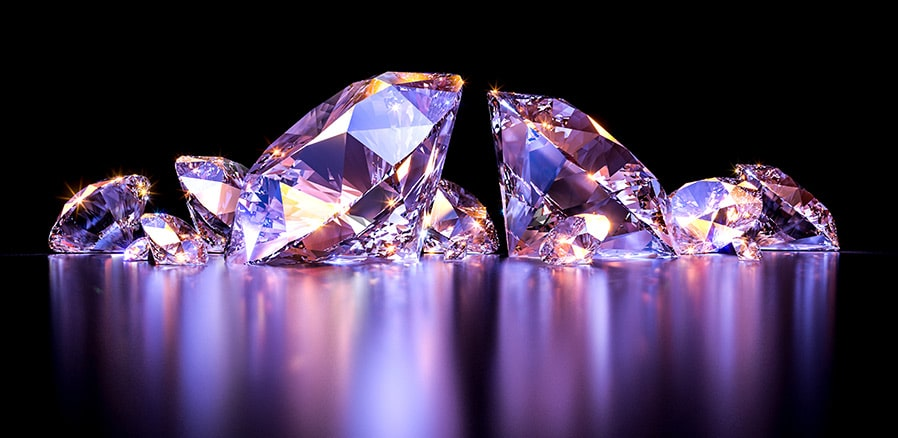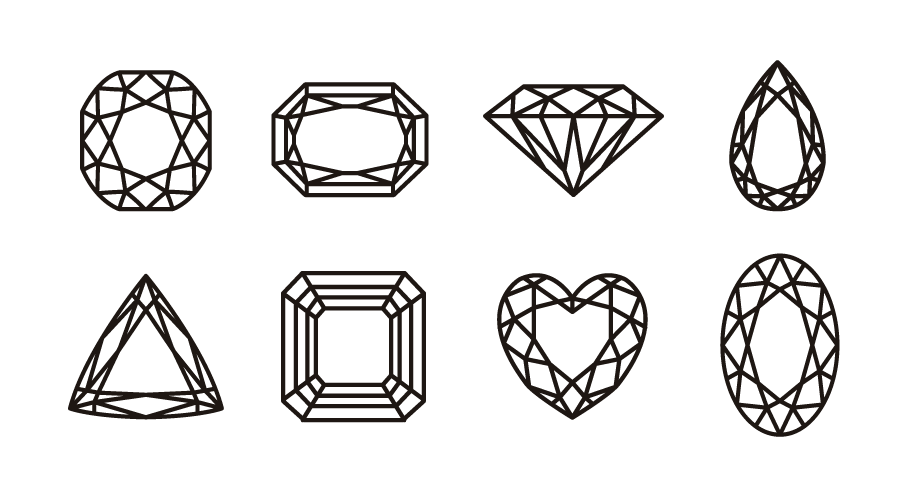# Diamond Weight Calculator

## Are you trying to find the perfect diamonds for an engagement ring?

The carat weight is a standard measure of how much a diamond weighs. It has a significant impact on diamond prices. Therefore, the diamond weight calculators and calculations formulas are here to help you calculate your gem's carat weight.

Choose from a dozen shapes, such as heart-shaped or pear-shaped diamonds, to find the one you require. Continue reading if you want to learn more about diamond weight formulas or what a diamond carat weight is. In addition, we are confident that you will find this ring-size converter useful.

Remember Marilyn Monroe's wise words. Diamonds are a girl's best friend. 💎### What is a carat of a diamond?

For the physical weight, the unit of measurement of brilliant diamonds is the carat. One carat is equal to 100 points divided by 1 and equals 0.200 grams or 1/5 gram. For comparison, one carat equals 0.007ounce in units more commonly used in the United States. Therefore, one pound would require over 2,265 carats.

Carat is a mass unit used to weigh gemstones and pearls. It got its name from carob tree seeds used for centuries to weigh gemstones. More than a century ago, the carat unit was standardized and is now equal to 200 mg.

• 1 carat = 200 mg = 0.2 g
• 1 carat = 0.007055 oz

### What is a good diamond weight?

Diamonds around the 0.70-ct mark are a nice compromise between size and price and make excellent engagement ring stones. A fine diamond of this weight can be obtained for around \$2,000.

### What's the 1-carat diamond price?

We cannot answer this question without updated data. However, the most obvious factor influencing the price of a gemstone is its weight in carats, and a diamond weight calculator can calculate the approximate weight of the selected size and shape gem.

However, you can't estimate the cost of a diamond ring on that basis because it's only one of the few factors influencing diamond value. The following are the essential diamond characteristics:

• Carat weight
• Cut and shape
• Clarity
• Colour
• Fluorescence

Remember that the price of brilliant diamonds does not increase proportionally to their weight; a 3-carat diamond will charge more than three 1-carat diamonds. Have you ever heard of the iconic Koh-i-Noor Diamond housed in the British Crown Jewels?

It is one of the world's largest cut diamonds, weighing 105.6 carats, and its value is predicted to be more than \$200 million. That is more than the cost of a hundred 1-carat diamonds or fifty 2-carat diamonds, which are the most popular choices for engagement ring diamonds.

### Diamond shapes

Diamonds exist in a variety of shapes and sizes. The stunning round diamond is the most popular shape, accounting for roughly 3/4 of all diamond sales. However, other fancy-shaped diamonds are becoming increasingly popular, as they are usually cheaper than an equivalent round-shaped Diamond.

### Diamond dimension

The Diamond's size is measured in millimeters (mm). These dimensions are roughly equivalent to carats. A 1-carat round diamond is typically 6.5 mm in diameter, while a 1.25-carat round diamond is 6.8 mm in diameter. A 1-carat diamond is typically 5.5 mm for square-cut diamonds such as princess, cushion, and Asscher, and a 1.25-carat diamond is 6 mm.

## How to calculate the diamond weight?

We're still undecided. Should we go with a heart-shaped or pear-shaped diamond?But, first, let's see what the weight of the Diamond we want is:

#### Select the diamond shape

You have a choice of twelve different shapes. For example, assume you want to determine the weight of a pear-shaped diamond.

#### Diamond's dimensions

If you've chosen a round diamond, mark its length, width, Depth, or diameter and Depth. For example, consider the pear shape diamond, which is 6 mm long, 4 mm wide, and has a depth (height) of 3 mm.

#### Check the ring girdle

Nothing needs to be changed; if it's thicker, you may want to add a few percent. Assume our good Diamond has a medium girdle, so type, for example, 2%.

#### Determine whether the diamond shape is standard

Because our pear-shaped Diamond has broad wings, we enter 5%.

#### Apply the formula to know the diamond weight in carats

In our example, it's 0.45 carats.

### Girdle thickness and diamond correction factors

Girdle thickness, as defined by the GIA Laboratory, represents the thinnest and thickest "valley" positions on the girdle of a round brilliant — that is, the thin area where the lower and upper half facets meet — about Diamond's average diameter.

The girdle is Diamond's outermost edge. This thickness is expressed as a percentage of the diameter (e.g., thin = 1.0%, medium = 3.0%, thick = 4.0%, etc...) or in words (such as Medium, Thick or Thin).

If you want to be even more precise in your diamond weight estimate, you can consider two factors influencing the final diamond weight, girdle thickness, and shape correction.

A girdle is Diamond's outermost edge. It is described in the rating scale or expressed as a percentage of the average diameter (thin, medium, thick, super thick, etc.). Add:

• 0% - 1% if the girdle is very thin or thin
• 1% - 3% if medium
• 3% - 6% for thick and very thick girdles
• >6% for extra thick

If the shape of your ring is not ideally regular and standard, you can make additional correction adjustments to the final weight formula. The following are the most commonly used adjustment factors for selected shape variations:

#### Wide or fat wings, squarish or high shoulders

• Pear: +1% to +5%
• Marquise, Oval, Heart: +1% to +10%

#### Large Culet

• All Shapes: +1% to +2%

#### Wide Corners

• Emerald: -1% to -5%

#### Pavilion Bulge

•   Emerald, Radiant & Trillion: +1% to +18%

Enter the value in the diamond weight calculator's correction factor box, and the calculator will consider those conditions when calculating the final diamond weight.

## Diamond weight estimation formulas

The Diamond Carat Weight Calculator uses the following weight estimation formulas:

Where GTF = Girdle Thickness Factor, WCF = Weight Correction Factor.### Round Brilliant

Approximate Estimated Carat Weight = Diameter2 × Depth × 0.0061 × GTF

### Oval

Approximate Estimated Carat Weight = Length × Width × Depth × 0.0062 × GTF × WCF

### Princess Cut

Approximate Estimated Carat Weight = Length × Width × Depth × 0.0083 × GTF × WCF

### Asscher Cut

Approximate Estimated Carat Weight = Length × Width × Depth × 0.0080 × GTF × WCF

### Cushion Cut

Approximate Estimated Carat Weight = Length × Width × Depth × 0.00815 × GTF × WCF

### Heart

Approximate Estimated Carat Weight = Length × Width × Depth × 0.0059 × GTF × WCF

### Baguette

Approximate Estimated Carat Weight = Length × Width × Depth × 0.00915 × GTF × WCF

### Emerald Cut

Approximate Estimated Carat Weight = Length × Width × Depth × Adjustment Factor × GTF × WCF

### Trillion Cut

Approximate Estimated Carat Weight = Length × Width × Depth × 0.0057 × GTF × WCF

 Length / Width Adjustment Factor 1 : 1 0.0080 1.5 : 1 0.0092 2 : 1 0.0100 2.5 : 1 0.0106

Approximate Estimated Carat Weight = Length × Width × Depth × Adjustment Factor × GTF × WCF

 Length / Width Adjustment Factor 1 : 1 0.0081 1.5 : 1 0.0084

### Marquise Cut

Approximate Estimated Carat Weight = Length × Width × Depth × Adjustment Factor × GTF × WCF

 Length / Width Adjustment Factor 1.5 : 1 0.00565 2 : 1 0.00580 2.5 : 1 0.00585 3 : 1 0.00595

### Pear

Approximate Estimated Carat Weight = Length × Width × Depth × Adjustment Factor × GTF × WCF

 Length / Width Adjustment Factor 1.25 : 1 0.00615 1.5 : 1 0.00600 1.66 : 1 0.00590 2 : 1 0.00575

### How can we calculate diamond weight?

The formulas are as follows: Length x Width x Depth x Coefficient = Diamond Carat. 5 x 3.5 x 2.5 x 0.0090 = 0.394 carats (approximately 39 satang) (points).

### What is the weight of a 1-carat diamond?

Carat is frequently confused with diamond size, even though it is a unit of weight. For example, one carat is now equal to 0.2 grams.

### How can we weigh diamonds at home?

To verify the carat weight of a diamond, weigh it and record the weight in grams. Then, divide that weight by 0.2 to get Diamond's weight in carats. For example, if a diamond weighs 0.1 grams, dividing it by 0.2 yields 0.5, indicating that the stone weighs half a carat.

### What is the worth of a 0.25-carat diamond?

A 0.25-carat diamond can be purchased for between \$275 and \$440. However, this price can change due to market factors.

### How can you notify if a diamond is genuine?

Place the stone, flat side down, on the dot. Look down onto the paper through the pointed or top end of the Diamond. If you see a circular reflection within the Diamond, it is a fake. The Diamond is genuine if you do not find a dot or reflection in the stone.

### Does a real diamond sparkle rainbow?

We all admire the way a stunning diamond sparkles in the sunlight. Put your stone in direct sunlight and observe the colors it reflects. A genuine diamond will reflect both rainbow colors and white light. If you only get one of them, the Diamond isn't genuine.

### Which carat diamond is best?

Diamonds cost more at 1.00 ct because it is the most popular weight. The price increase is especially pronounced for round diamonds; choosing a fancy shape at this weight will save you the most money.

### Which is the best Diamond?

Flawless is the highest clarity grade. Even when magnified 10x by a professional, an FL diamond has no flaws. The highest color grade is D, which stands for completely colorless Diamond. While flawless and colorless diamonds are rare, the cut of a diamond determines its true value.